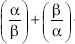# User Forum

Subject :IMO    Class : Class 10

For what value of a, the roots of the equation 2x2 + 6x + a = 0, satisfy the condition<2 (where α and β are the roots of equation).
A a < 0
B –1 < a < 0
C –1 < a < 1
D None of these